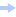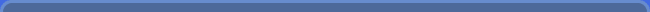Navigation: LaesieWorksIdentified Flying ObjectsGravityGRAVITY Here on the surface of the Earth, we are constantly under the influence of 1G of gravity. We don't think about that much, it's always like that. A force of 1G is very rare though. At most places in this universe there is less than 1G, and at some places there's more than 1G. 1G is just a point on a scale that runs from zero to incredible high. I don't know if there's an upper limit. Gravity; the ultimate propulsion type? Look at gravity sideways. Dropping a stone from a skyscraper. The stone accelerates very fast, but without a noisy fuel consuming fire spitting engine. For the stone, this acceleration is actually a relief of pressure. If there would be no air, the stone would be weightless. It's the most clean safe and efficient propulsion available, and it free. The trouble with natural gravity, is that it's always going down to earth, while we would like to fly with it, point it in a direction of choice. Gravity works in space, and not having to take propellant mass onboard would be a bless. But for that to happen, we first have to understand gravity. What is gravity? We don't know, not yet. We can calculate the forces, but don't really know the origin of it. Not yet. I find it embarrassing how little we know, and how little effort is put in discovering it. A "thank you" to the few people who do search to find out. What we can observe? - Atoms (objects with mass) have or cause gravity. - All G force of an object with mass, is directed towards its center. - Objects with mass, get forced together (attracted or pushed?). - The more distance from a mass, the weaker the force is. - Gravity can bent the direction of light. - Gravity slows down the speed of time (by influencing the condition of space?) - Heavy objects accelerate as fast by gravity as light objects (when there's no air or other resistance). The size of gravity How far can a gravity force reach? Indefinitely, or for a certain distance? Curved Space? Is the Earth turning around the sun, constantly freefalling towards the Sun, or is the space-time around the sun curved and the path the Earth's following actually straight? "Geodesics" The path of the Earth around the sun, is probably the path of least resistance. A balance between gravity and relative speed. Straight through atoms I know of no material that can schield or reflect this force. It passes through atoms, with no loss? The speed of gravity If the sun would suddenly disappear, how long would it take before that has its effect on the path of the Earth? Would the effect be instantly, or would there be a delay? If there is a delay, will the speed of gravity be equal that of light? Infinite speed, that seems to be out of the question, but then again; maybe not. "Quantum entanglement" is about the connection two split particles can have. When touching the one half of the entangled pair, it has an instant effect on the other half particle, no matter how great a distance in between. If this new phenomena is real, then an instant effect of gravity could be true also. The are trying to measure gravitational waves, but have detected none so far. It has been indirectly shown to exist. A weak force, that can be very strong. Two marbles don't accelerate towards each other fast. The force of gravity for each atom is weak, relative to for example the strong forces that keep molecules together. It takes the immense mass of the Earth to produce just 1G. The more mass, the more gravity. All the atoms gravity forces combine together as one. The Earth's gravitational field might not be strong relative to other forces, but a gravitational field can be very strong indeed, and it's spread over a very large area of space. Black holes (with are sferes actually, if they exist) have so much G force that atoms collapse and light can't escape from it. Compared to pressurized gas Does the presence of mass decrease the density of space? You know how a bar of soap can shoot away when you squeeze it.. kind of like that. Is space an "Ether" medium after all? Does space support the presence of gravity particles, or is gravity a change in the pressure of space? A balloon has a force up because he ambient air has more mass per volume, pushing the balloon away from the bottom. If there would be no gravity, the balloon would not rise. Could the pressure of space be less towards the center of gravity? More dense space pushing less dense atoms away from space? Do the Sun and Earth attract each other, or is space pushing them together? Compared to a magnetic field A gravitational field is not equal to a magnetic field, but there are some similarities. Both fields are static, so it seems. There is no north and south pole like magnets have. A differences of field force doesn't create a flow, like air would do. To create an electric magnetic field, one needs to put energy in it, and as a magnetic field collapses, energy comes free. I imagine one has to put energy in space to create a gravitational field also, and that energy will come free when if the field collapses. Creating gravity We know that an extremely large mass has or causes gravity. Like stars and planets. We don't know how to create gravity any other way. But we would like to! Acceleration Acceleration is interesting. When accelerating an object with mass, this object experiences a force that is equal to gravity, even while it's not in a field of gravity. Accelerating an object with 9.8 m/s2 is setting 1G on it, exactly the same as on the surface of the Earth. A turning wheel its atoms are in constant acceleration. A way to produce "artificial gravity". This is one of reasons why the disc shape is so fit for space travel. But a spinning wheel doesn't create a field of gravity, and the acceleration forces point in opposite directions, giving zero thrust total. A mass in a gravitational field is experiencing a force equal to accelerating. Is it exactly the same, or not? 1G is making atoms accelerate by 9.8 m/s2 (if there's no resistance). An object near the Earth, falling towards the Earth: After 1 second, it's velocity relative to the Earth = 9.8 m/s After 2 seconds = 19.6 m/s After 3 seconds = 29.4 m/s After 4 seconds = 39.2 m/s This object is in "freefall" and does NOT experience the presence of the 1G environment. It's mass weighs nothing. It's as much at rest as if it would be in empty space. And if this object would be in empty space, and be accelerated to 1G by a rocket, the object would experience exactly the same fore as when standing still on the surface of the Earth. So, I might be wrong, but it seems like (taking about force only): Standing still on Earth = Being accelerated by a rocket in empty space. Falling to Earth = Being in empty space.

 Giesbert